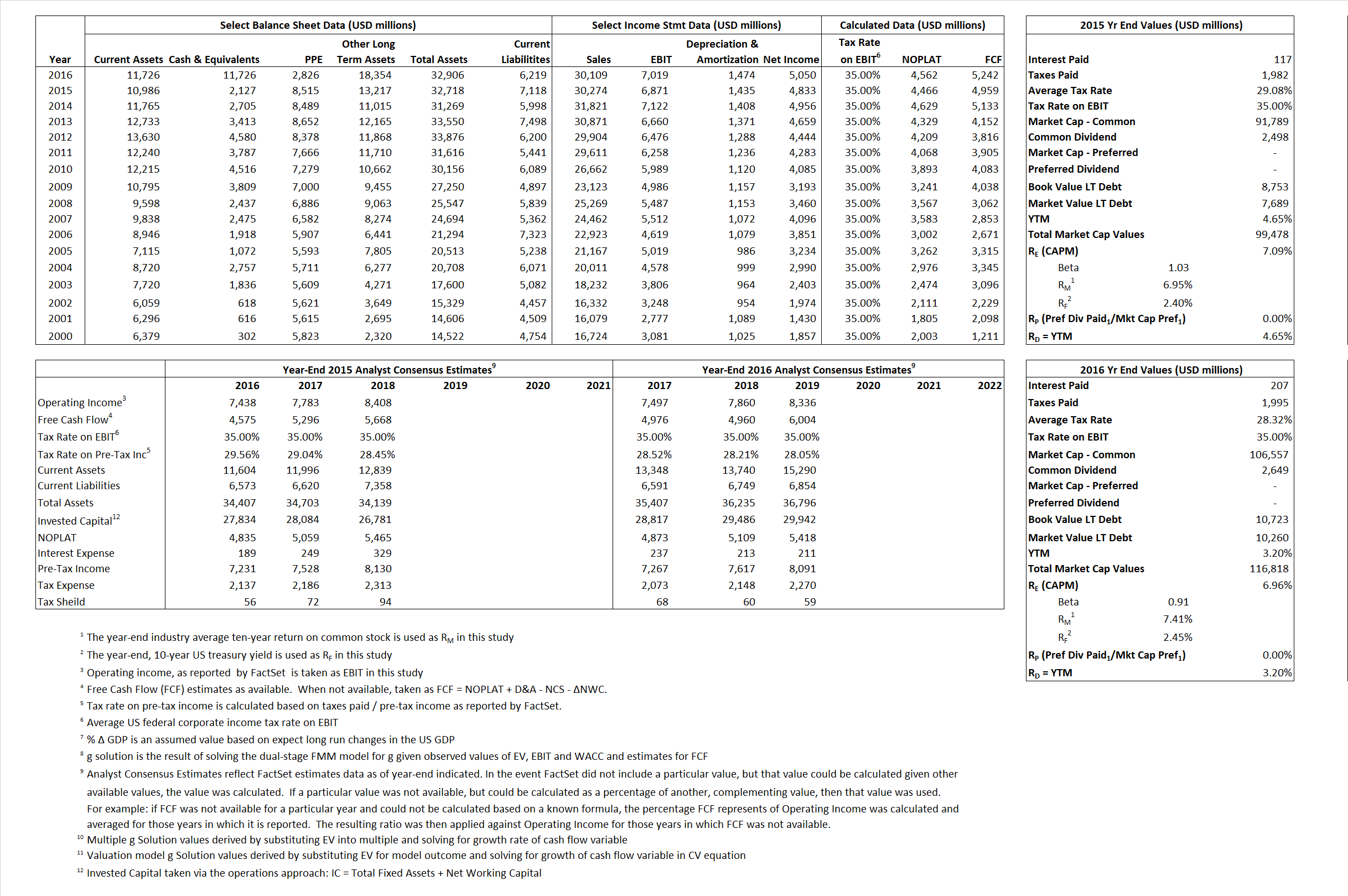# Minnesota Mining & Manufacturing (3M)

## Analyst Listing

The following analysts provide coverage for the subject firm as of May 2016:

 Broker Analyst Analyst Email Morningstar Barbara Noverini barbara.noverini@morningstar.com Langenberg, LLC Brian K. Langenberg brian@langenberg-llc.com RBC Capital Markets Deane M. Dray deane.dray@rbccm.com Argus Research John Eade jeade@argusresearch.com Deutsche Bank Research John G. Inch john.inch@db.com Credit Suisse Julian Mitchell julian.mitchell@credit-suisse.com Jefferies Laurence Alexander lalexander@jefferies.com Erste Group Martin Krajhanzl mkrajhanzl@csas.cz William Blair Nicholas P. Heymann nheymann@williamblair.com Stifel Nicolaus Robert P. McCarthy mccarthyr@stifel.com Hilliard Lyons Spencer E. Joyce sjoyce@hilliard.com Bernstein Research Steven E. Winoker steven.winoker@bernstein.com Stifel Nicolaus Robert P. McCarthy mccarthyr@stifel.com Bernstein Research Steven E. Winoker steven.winoker@bernstein.com Langenberg, LLC Brian K. Langenberg brian@langenberg-llc.com Deutsche Bank Research John G. Inch john.inch@db.com RBC Capital Markets Deane M. Dray deane.dray@rbccm.com Hilliard Lyons Spencer E. Joyce sjoyce@hilliard.com Jefferies Laurence Alexander lalexander@jefferies.com William Blair Nicholas P. Heymann nheymann@williamblair.com Credit Suisse Julian Mitchell julian.mitchell@credit-suisse.com

## Primary Input Data## Derived Input Data

### Equational Form

Net Operating Profit Less Adjusted Taxes NOPLAT  4,466 4,562$NOPLAT\, =\, EBIT\, x\, (1 \,-\, Avg \,\,Tax\,\, Rate\,\, on\,\, EBIT)$
Free Cash Flow FCF  4,959  5,242$FCF\,=NOPLAT\,+\,Non-Cash\,Expenses-\Delta NWC\,-\,NCS$
Tax Shield TS 34  59$TS\,=\,Interest\,\,Paid\,\,x\,\, Avg \,\,Tax\,\,Rate\,\, on\,\, Pre-Tax\,\, Income$
Invested Capital IC 25,600 26,687$IC\,=\,Fixed\,\,Operating\,\,Assets\,\,+\,\,Net\,\, Working\,\, Capital$
Return on Invested Capital ROIC 17.45% 17.10%$ROIC\,=\,\frac { NOPLAT }{ IC }$
Net Investment NetInv 1,764 2,561$NetInv\,=\,{ {IC}_{1}}-{{IC}_{0}}+Depreciation$
Investment Rate IR 39.50%  56.13%$IR\,=\,\frac {NetInv}{NOPLAT}$
Weighted Average Cost of Capital
WACCMarket 6.79%  6.55%$WACC\,=\,\frac { E }{ V } { R }_{ E }\,+\,\frac { P }{ V } { R }_{ P }\,+\,\frac { D }{ V } { R }_{ D }\left( 1- Avg\,\, Tax\,\,Rate\,\,on\,\,Pre-Tax\,\,Income \right)$
WACCBook  8.76%   7.77%
Enterprise value
EVMarket 97,351  105,092$EV\,=\,Market\,\,Cap\,\,Equity\,+\,\,Long\,\,Term\,\,Debt\,-\,Cash$
EVBook  88,816  105,554
Long-Run Growth
g = IR x ROIC
6.89%  9.60% Long-run growth rates of the income variable are used in the Continuing Value portion of the valuation models.
g = %$\Delta$ GDP   2.50%   2.50%
Margin from Operations M  22.70%  23.31%$M\,\,=\,\,\frac{EBIT}{SALES}$
Depreciation/Amortization Rate D  17.28%  17.36%$D\,\,=\,\,\frac{D+A}{EBITDA}$

## Valuation Multiple Outcomes

The outcomes presented in this study are the result of original input data, derived data, and synthesized inputs.

### model g solution

12/31/2015 12/31/2016 12/31/2015 12/31/2016 12/31/2015 12/31/2016

EV/SALES$\frac {EV}{Sales} \,= \,\frac{ROIC\, -\, g}{ROIC\,(WACC\,-\,g)}\,(1\,-\,T)\,(M)$

3.22  3.49  2.99%  2.97%  3.23%  3.75%

EV/EBITDA$\frac {EV}{EBITDA} \,= \,\frac{ROIC\, -\, g}{ROIC\,(WACC\,-\,g)}\,(1\,-\,T)\,(1\,-\,D)$

11.72 12.37 2.99% 2.97% 3.23% 3.75%

EV/NOPLAT$\frac {EV}{NOPLAT} \,= \,\frac{ROIC\, -\, g}{ROIC\,(WACC\,-\,g)}$

21.80 23.03 2.99% 2.97% 3.23% 3.75%

EV/FCFOPS$\frac {EV}{FCF_{OPS}} \,= \,\frac{ROIC\, -\, g}{ROIC\,(WACC\,-\,g)}\,(1\,-\,T)$

19.63 20.05 2.99% 2.97% 3.23% 3.75%

EV/EBIT$\frac {EV}{EBIT} \,= \,\frac{ROIC\, -\, g}{ROIC\,(WACC\,-\,g)}\,(1\,-\,T)$

14.17 14.97 2.99% 2.97% 3.23%  3.75%

EV/IC$\frac {EV}{IC} \,= \,\frac{ROIC\, -\, g}{WACC\,-\,g}$

3.80 3.94 2.99% 2.97% 3.23%  3.75%